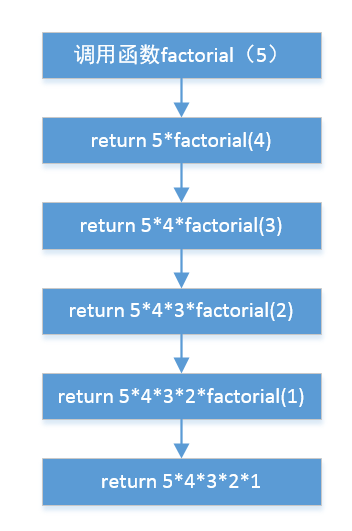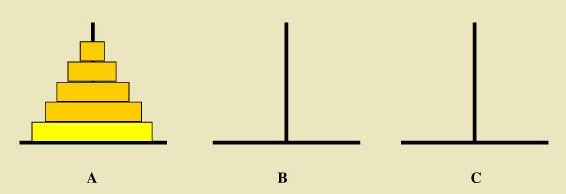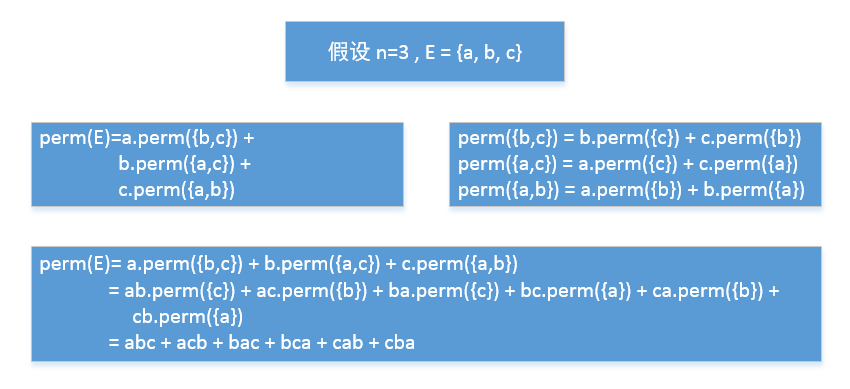# 递归算法 汉诺塔 排列组合

a>子问题与原始问题为同样的事，且更为简单；

b>不能无限制的调用自身，必须有一个出口，化简为非递归状况处理。

int factorial(int n){
if (n == 1)
return 1;
else
return n*factorial(n - 1);
}(1)  n==1

第1次 A ---> C   共1次移动

(2)  n==2

第1次 A ---> B

第2次 A ---> C

第3次 B ---> C  共3次移动

(3)  n==3

第1次  A ---> C

第2次 A ---> B

第3次 C ---> B

第4次  A ---> C

第5次 B ---> A

第6次 B ---> C

第7次 A ---> C 共7次移动

void move(int plateNum, char F, char T)
{
cout << F << " ---> " << T << endl;
}

// num:圆盘数量  A B C 分别代表三棵柱子
void hanio(int num, char A, char B, char C)
{
if (num == 1)
move(1, A, C);
else
{
hanio(num - 1, A, C, B);
move(num, A, C);
hanio(num - 1, B, A, C);
}
}#pragma once

#include <iostream>
using namespace std;

template<class T>
void Perm(T list[], int k, int m)
{
int i;
if (k == m)
{
for (i = 0; i <= m; i++)
cout << list[i];
cout << endl;
}
else
{
for (i = k; i <= m; i++)
{
Swap(list[k], list[i]);
Perm(list, k+1, m);
Swap(list[k], list[i]);
}
}
}

template<class T>
inline void Swap(T&a, T&b)
{
T temp = a;
a = b;
b = temp;
}

int _tmain(int argc, _TCHAR* argv[])
{
int list = { 1, 2, 3 };
Perm(list, 0, 2);

return 0;
}

02-171097
10-13217609-143210
06-024105
11-301163
03-1869
05-011万+
03-11688
04-294万+
08-277457